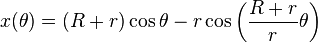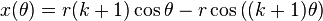##In geometry, an epicycloid is a plane curve produced by tracing the path of a chosen point of a circle — called an epicycle — which rolls without slipping around a fixed circle. It is a particular kind of roulette.

If the smaller circle has radius r, and the larger circle has radius R = kr, then the parametric equations for the curve can be given by either:$x (\theta) = (R + r) \cos \theta - r \cos \left( \frac{R + r}{r} \theta \right)$$y (\theta) = (R + r) \sin \theta - r \sin \left( \frac{R + r}{r} \theta \right),$

or:$x (\theta) = r (k + 1) \cos \theta - r \cos \left( (k + 1) \theta \right) \,$$y (\theta) = r (k + 1) \sin \theta - r \sin \left( (k + 1) \theta \right). \,$

If k is an integer, then the curve is closed, and has k cusps (i.e., sharp corners, where the curve is not differentiable).

If k is a rational number, say k=p/q expressed in simplest terms, then the curve has p cusps.

If k is an irrational number, then the curve never closes, and fills the space between the larger circle and a circle of radius R + 2r.

wikipedia

via:

https://clearcircle.wordpress.com/page/2/

This entry was posted in Body. Bookmark the permalink.